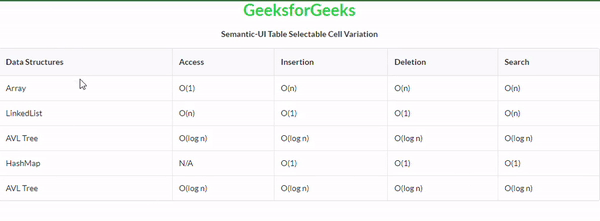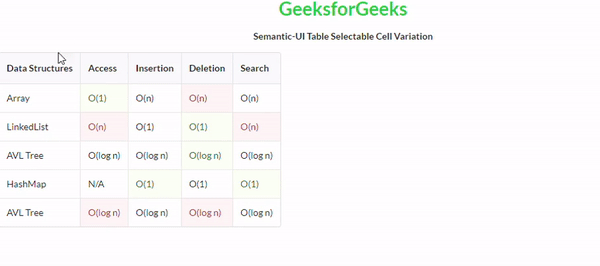Open in App
Not now

# Semantic-UI Table Selectable Cell Variation

• Last Updated : 24 Mar, 2022

Semantic UI is an open-source framework that uses CSS and jQuery to build great user interfaces. It is the same as a bootstrap for use and has great different elements to use to make your website look more amazing. It uses a class to add CSS to the elements.

Tables are an easy way to organize a lot of data. A table is an arrangement of data in rows and columns, or possibly in a more complex structure. Tables are widely used in communication, research, and data analysis. Tables are useful for various tasks such as presenting text information and numerical data. It can be used to compare two or more items in the tabular form layout. Tables are used to create databases. An HTML table and a Semantic UI table both are the same structurally.

In the case of Selectable Cell variation, we can make a table cell selectable, we do this by using anchor tags which enable the cell to be selectable, and also these links by default will inherit their cell color.

Semantic UI Table Selectable Cell Variation Class:

• selectable: This class enables a cell of the table to be selectable.

Syntax:

```<table class="ui table">
<tr>
<td class="selectable"></td>
...
</tr>
...
</table>```

Example 1: This is a basic example illustrating Table Selectable Cell variation created using Semantic UI.

## HTML

 ` ` `<``html``> ` `    ``<``head``> ` `        ``<``title``>Semantic-UI Table Selectable Cell Variation ` `        ``<``link` `href``= ` `"https://cdnjs.cloudflare.com/ajax/libs/semantic-ui/2.4.1/semantic.min.css"`  `             ``rel``=``"stylesheet"` `/>       ` `    `` ` `    ``<``body``> ` `        ``<``center``> ` `            ``<``h1` `class``=``"ui green header"``>GeeksforGeeks ` `            ``<``strong``>Semantic-UI Table Selectable Cell Variation ` `        `` ` `        ``<``table` `class``=``"ui celled table"``> ` `            ``<``thead``> ` `                ``<``tr``> ` `                    ``<``th``>Data Structures ` `                    ``<``th``>Access ` `                    ``<``th``>Insertion ` `                    ``<``th``>Deletion ` `                    ``<``th``>Search ` `                `` ` `            `` ` `            ``<``tbody``> ` `              ``<``tr``> ` `                ``<``td``>Array ` `                ``<``td` `class``=``"selectable"``><``a``>O(1) ` `                ``<``td``>O(n) ` `                ``<``td``>O(n) ` `                ``<``td``>O(n) ` `              `` ` `              ``<``tr``> ` `                ``<``td``>LinkedList ` `                ``<``td``>O(n) ` `                ``<``td` `class``=``"selectable"``><``a``>O(1) ` `                ``<``td``>O(1) ` `                ``<``td``>O(n) ` `              `` ` `              ``<``tr``> ` `                ``<``td``>AVL Tree ` `                ``<``td``>O(log n) ` `                ``<``td``>O(log n) ` `                ``<``td` `class``=``"selectable"``><``a``>O(log n) ` `                ``<``td``>O(log n) ` `              `` ` `              ``<``tr``> ` `                ``<``td``>HashMap ` `                ``<``td``>N/A ` `                ``<``td``>O(1) ` `                ``<``td``>O(1) ` `                ``<``td` `class``=``"selectable"``><``a``>O(1) ` `              `` ` `              ``<``tr``> ` `                ``<``td``>AVL Tree ` `                ``<``td` `class``=``"selectable"``><``a``>O(log n) ` `                ``<``td``>O(log n) ` `                ``<``td``>O(log n) ` `                ``<``td``>O(log n) ` `              `` ` `          `` ` `        `` ` `    `` ` ``

Output:Example 2: This is a basic example illustrating Collapsing Table Selectable Cell variation created using Semantic UI.

## HTML

 ` ` `<``html``> ` `    ``<``head``> ` `        ``<``title``>Semantic-UI Table Selectable Cell Variation ` `        ``<``link` `href``= ` `"https://cdnjs.cloudflare.com/ajax/libs/semantic-ui/2.4.1/semantic.min.css"`  `             ``rel``=``"stylesheet"` `/>        ` `    `` ` `    ``<``body``> ` `        ``<``center``> ` `            ``<``h1` `class``=``"ui green header"``>GeeksforGeeks ` `            ``<``strong``>Semantic-UI Table Selectable Cell Variation ` `        `` ` `        ``<``table` `class``=``"ui celled table collapsing"``> ` `            ``<``thead``> ` `                ``<``tr``> ` `                    ``<``th``>Data Structures ` `                    ``<``th``>Access ` `                    ``<``th``>Insertion ` `                    ``<``th``>Deletion ` `                    ``<``th``>Search ` `                `` ` `            `` ` `            ``<``tbody``> ` `              ``<``tr``> ` `                ``<``td``>Array ` `                ``<``td` `class``=``"selectable positive"``><``a``>O(1) ` `                ``<``td``>O(n) ` `                ``<``td` `class``=``"negative"``>O(n) ` `                ``<``td``>O(n) ` `              `` ` `              ``<``tr``> ` `                ``<``td``>LinkedList ` `                ``<``td` `class``=``"negative"``>O(n) ` `                ``<``td` `class``=``"selectable"``><``a``>O(1) ` `                ``<``td` `class``=``"positive"``>O(1) ` `                ``<``td` `class``=``"negative"``>O(n) ` `              `` ` `              ``<``tr``> ` `                ``<``td``>AVL Tree ` `                ``<``td``>O(log n) ` `                ``<``td``>O(log n) ` `                ``<``td` `class``=``"selectable positive"``><``a``>O(log n) ` `                ``<``td``>O(log n) ` `              `` ` `              ``<``tr``> ` `                ``<``td``>HashMap ` `                ``<``td``>N/A ` `                ``<``td` `class``=``"positive"``>O(1) ` `                ``<``td``>O(1) ` `                ``<``td` `class``=``"selectable positive"``><``a``>O(1) ` `              `` ` `              ``<``tr``> ` `                ``<``td``>AVL Tree ` `                ``<``td` `class``=``"selectable negative"``><``a``>O(log n) ` `                ``<``td``>O(log n) ` `                ``<``td` `class``=``"negative"``>O(log n) ` `                ``<``td``>O(log n) ` `              `` ` `          `` ` `        `` ` `    `` ` ``

Output:My Personal Notes arrow_drop_up
Related Articles